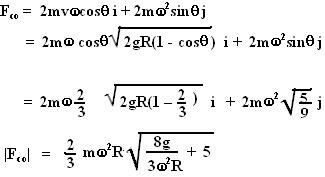## Wednesday, February 13, 2008

### Irodov Problem 1.115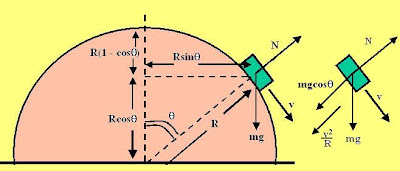First let us start looking the problem from a stationary frame (a non-rotating frame). There are two forces i) acting on the mass, i) the normal reaction from the surface N and the force of gravity mg as shown in the figure. Let us consider the forces acting in a direction normal to the surface of the sphere. There are two forces acting in this direction, N and. The body is subject to centrifugal accelerationof as it moves (slides) along the curvature of the sphere in a circular path. Thus we have,As the body slides down it looses potential energy and gains speed (kinetic energy). When it is at an angle, the mass has fallen a height of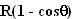and looses a potential energy of. This will get converted into kinetic energy of the body, hence we have,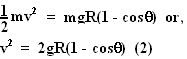At the break-off point, the normal reaction N offered by the surface becomes 0. From (1) and (2) and setting N=0 in (1) we have,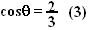Now coming to the problem. The centrifugal force seen by the observer in the rotating frame is given by(see here). Here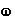is the angular velocity vector of the rotating frame as seen from a stationary reference and r is the radius vector of the particle as shown in the figure in the rotating reference . For this problem,Thus, the centrifugal force experienced by the mass in the rotating frame is.The Coriolis force is defined as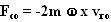(see here.) Hereis the angular velocity of the rotating frame of reference with respect to a stationary reference andis the velocity of the mass relative to the rotating frame.
Relative to the rotating frame the mass's velocity is a sum of two motions, i) the tangential velocity of the mass on the surface of the sphere as it slides down v and ii) the apparent rotation of the mass along the horizontal plane with angular velocityin the opposite direction to the rotation of the sphere (basically to someone on the sphere the mass rotates with an angular velocityin the opposite direction). This is depicted in the picture.If we write the velocity vector as,as shown in the figure beside and the angular velocity ask then the Coriolis force can be determined as,